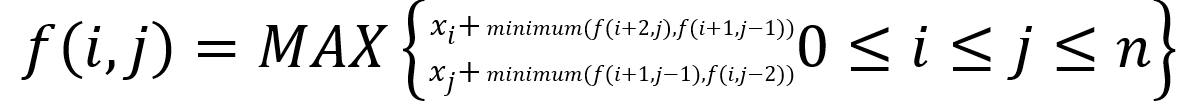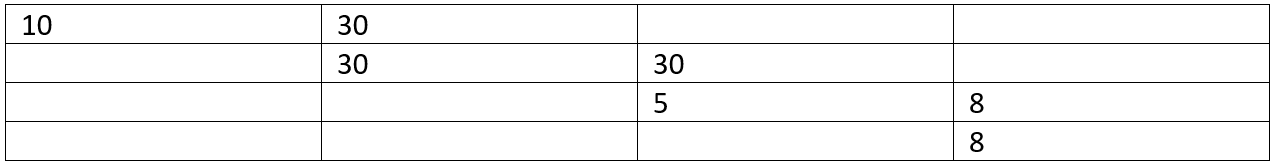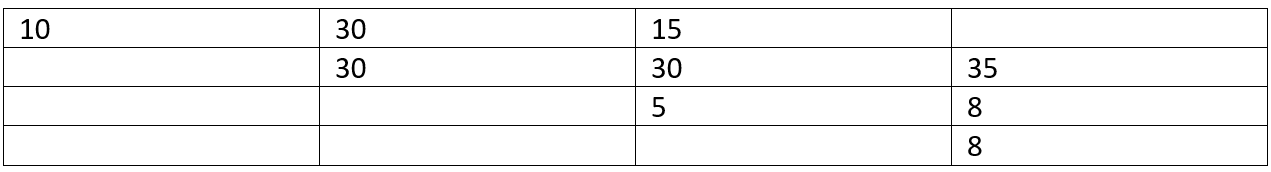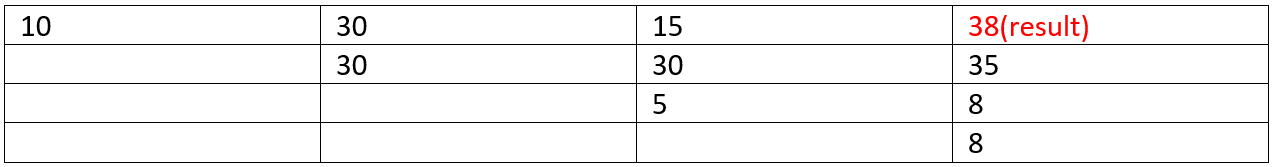# Optimal Strategy for a Game

Optimal Strategy for a Game: Here, we are going to learn to find maximum outcomes in a two player game played in an optimal way.
Submitted by Radib Kar, on February 03, 2020

Description:

This is a very popular interview problem to find maximum outcomes in a two player game played in an optimal way. This problem has been featured in interview rounds of Amazon, Microsoft, Salesforce.

Problem statement:

You are given an array A of size N. The array contains integers and is of even length. The elements of the array represent N coin of values x1, x2, ... ,xn. The rule of game is:

1. Each of the players gets alternative turns.
2. In each turn, a player selects either the first or last coin from the row, removes it
3. permanently, and receives the value of the coin.
4. Both the player plays in an optimal way, i.e., both want to maximize total winning money.

You need to determine the maximum possible amount of money you can win if you start the game.

```    Input
N=4
Array values:
10 30 5 8

Output:
38
```

Example:

```    Number of coins: 4
Coin values:
10 30 5 8

To achieve maximum money:
I will pick up 8 first (Not 10. Think Why?)
So the row will be:
10 30 5
The opponent will pick 10 as that's optimal
So the row will be:
30 5
I will pick 30
Opponent will get 5

Total my winnings: 8+30=38
Total opponents winning: 10+5=15
```

Explanation:

The first thing that comes to our mind that we should go for the greedy method. Since we can only pick either first element or last element, let's pick the local best one, i.e., Pick the one which is maximum of first and last element. Let's see whether such a method would be optimal or not.

Let's take the same example,

```    Coin values:
10 30 5 8

To achieve maximum money using greedy approach:
I will pick up 10 first (maximum of 10 and 8)
So the row will be:
30 5 8
The opponent will pick 30 as that's maximum of 30 and 8
So the row will be:
5 8
I will pick 8
Opponent will get 5

Total my winnings: 10+8=18
Total opponents winning: 30+5=35
```

Is this optimal? I got less money than the opponent. So we can simply choose the local best one ( Answer of Why), we can't go for greedy.

Let's check what can be optimal strategy,

Say the coins are,

```    x1, x2, ..., xi, x(i+1), ..., x(j-1), x(j), ..., xn
```

Let,

```    f(i,j)=maximum wiining for me when ith to jth coins are remaining
```

There at any stage of game
And it's my turn
So, I can either pick xi or xj based on the optimal choice.

1. So If I choose xi , opponent will pick either of x(i+1) or xj leaving me with minimum(f(i+2,j),f(i+1,j-1))
```
Opponent choosing x(i+1) corresponds to f(i+2,j)
Opponent choosing xj corresponds to f(i+1,j-1)
```
2. If I choose xj , opponent will pick either of xi or x(j-1) leaving me with minimum(f(i+1,j-1),f(i,j-2))
```    Opponent choosing xi corresponds to f(i+1,j-1)
Opponent choosing x(j-1) corresponds to f(i,j-2)
```

So, I will choose xi or xj what gives me maximum.
so,Above is the recursion and we need to convert it into Dynamic Programming.

Problem Solution:

We need a 2D array to store f(i,j)

Say, DP[n][n] and the result will be DP[n-1] //maximum winning for coin 0th to nth (all the coins)

Base case:

```    If there is only one coin left, there's no choice
f(i,i)=arr[i] // arr is the array storing coin values in the row
So,
for i=0 to n-1
DP[i][i]=arr[i]
END for

If there is only two coin, i.e., xi, x(i+1)
I will choose the maximum obviously for maximum winning
for i=0 to n-2
DP[i][i+1]=arr[i]
END for
```

To fill rest of the Table using the recursion,

```    for (int len = 2; len < n; len++) {
for (int i = 0, j = len; j < n; i++, j++) {
DP[i][j] = max⁡(a[i] + min⁡(DP[i + 2][j], DP[i + 1][j - 1]),
a[j] + min(DP[i + 1][j - 1], DP[i][j - 2]));
}
}
```

Initial DP table after computing base cases

DP // N=4Filling up the upper triangular matrix

Len=2Len=3C++ implementation:

```#include <bits/stdc++.h>
using namespace std;

int max(int x, int y)
{
if (x > y)
return x;
else
return y;
}
int min(int x, int y)
{
if (x < y)
return x;
else
return y;
}
int MAX_WINNING(vector<int> a, int n)
{

int DP[n][n]; //DP matrix
memset(DP, 0, sizeof(DP));
//base case
for (int i = 0; i < n; i++) {
DP[i][i] = a[i];
if (i != n - 1) {
DP[i][i + 1] = (a[i] > a[i + 1]) ? a[i] : a[i + 1];
}
}
//filling up table using recusrion
for (int len = 2; len < n; len++) {
for (int i = 0, j = len; j < n; i++, j++) {
DP[i][j] = max(a[i] + min(DP[i + 2][j], DP[i + 1][j - 1]), a[j] + min(DP[i + 1][j - 1], DP[i][j - 2]));
}
}
//return result
return DP[n - 1];
}
int main()
{
int n, item;
cout << "Enter number of Coins\n";
scanf("%d", &n);
cout << "Enter coin values\n";
vector<int> arr;
for (int j = 0; j < n; j++) {
scanf("%d", &item);
arr.push_back(item);
}

cout << "Maximum possible winning is: " << MAX_WINNING(arr, n) << endl;

return 0;
}
```

Output

```Enter number of Coins
4
Enter coin values
10 30 5 8
Maximum possible winning is: 38
```